VML Reference Material - VML

This element specifies semantic information for a limited set of structured diagrams that have VML representations. Note that diagrams should be defined using DrawingML; this representation is included for compatibility with applications that rely on VML. The following diagram types have VML representations:

 Diagram Type Example (non-normative) Organization chart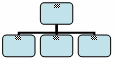Radial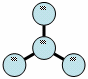CyclePyramid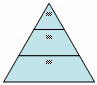Venn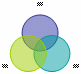Bulls-eye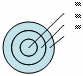Each of these types of diagrams contains shapes that are positioned relative to one another. Each shape also has optional associated text.

 Parent Elements background (§2.2.1); group (§6.1.2.7); hdrShapeDefaults (§2.15.1.50); object (§2.3.3.19); pict (§2.3.3.21); pict (§2.9.23); shapeDefaults (§2.15.1.79)

 Child Elements Subclause relationtable (Diagram Relationship Table) §6.2.2.24

 Attributes Description autoformat (Diagram Automatic Format) Specifies whether the diagram is formatted automatically by the application and user overrides are locked. Default is false.   [Example:     end example]   The possible values for this attribute are defined by the ST_TrueFalse simple type (§6.2.3.23). autolayout (Diagram Automatic Layout) Specifies whether the diagram elements are laid out automatically by the application and user overrides are locked. Default is true.   [Example:     end example]   The possible values for this attribute are defined by the ST_TrueFalse simple type (§6.2.3.23). constrainbounds (Diagram Layout Extents) Specifies an optional, application-specific parameter related to the diagram's extents intended to be used by the application to assist laying out the diagram.   [Example:     end example]   The possible values for this attribute are defined by the XML Schema string datatype. dgmbasetextscale (Diagram Base Font Size) Specifies the diagram's original font size. This is used in subsequent font size recalculations. If the most recent diagram font size is used to calculate the font size after a rescale, the font size would be wrong after non-isometric diagram rescalings.   [Example:     end example]   The possible values for this attribute are defined by the XML Schema integer datatype. dgmfontsize (Diagram Font Size) Specifies the font size for attached text when a new diagram node is added.   [Example:     end example]   The possible values for this attribute are defined by the XML Schema integer datatype. dgmscalex (Diagram Layout X Scale) Specifies an optional, application-specific parameter related to the horizontal scaling of the diagram that is intended to be used by the application to assist laying out the diagram.   [Example:     end example]   The possible values for this attribute are defined by the XML Schema integer datatype. dgmscaley (Diagram Layout Y Scale) Specifies an optional, application-specific parameter related to the vertical scaling of the diagram that is intended to be used by the application to assist laying out the diagram.   [Example:     end example]   The possible values for this attribute are defined by the XML Schema integer datatype. dgmstyle (Diagram Style Options) Specifies an optional, application-specific parameter related to the styling of the diagram that is intended to be used by the application to assist in formatting the diagram.   [Example:     end example]   The possible values for this attribute are defined by the XML Schema integer datatype. ext (VML Extension Handling Behavior)   Namespace: urn:schemas-microsoft-com:vml Specifies an optional value that indicates how applications that implement VML should interpret extensions not defined as part of the original specification of core VML.   [Rationale: This part of the original VML specification is included to assist applications that leverage existing VML support in implementing the Office Open XML Format. end rationale]   The possible values for this attribute are defined by the ST_Ext simple type (§6.1.3.3). reverse (Diagram Reverse Direction) Specifies whether the order of the diagram nodes is reversed. This is only relevant to diagrams that have linear ordering.   [Example: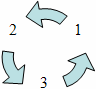reverse="false"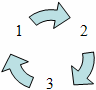reverse="true" end example   The possible values for this attribute are defined by the ST_TrueFalse simple type (§6.2.3.23).

The following XML Schema fragment defines the contents of this element:

<complexType name="CT_Diagram">

<sequence>

<element name="relationtable" type="CT_RelationTable" minOccurs="0"/>

</sequence>

<attributeGroup ref="v:AG_Ext"/>

<attribute name="dgmstyle" type="xsd:integer" use="optional"/>

<attribute name="autoformat" type="ST_TrueFalse" use="optional"/>

<attribute name="reverse" type="ST_TrueFalse" use="optional"/>

<attribute name="autolayout" type="ST_TrueFalse" use="optional"/>

<attribute name="dgmscalex" type="xsd:integer" use="optional"/>

<attribute name="dgmscaley" type="xsd:integer" use="optional"/>

<attribute name="dgmfontsize" type="xsd:integer" use="optional"/>

<attribute name="constrainbounds" type="xsd:string" use="optional"/>

<attribute name="dgmbasetextscale" type="xsd:integer" use="optional"/>

</complexType>